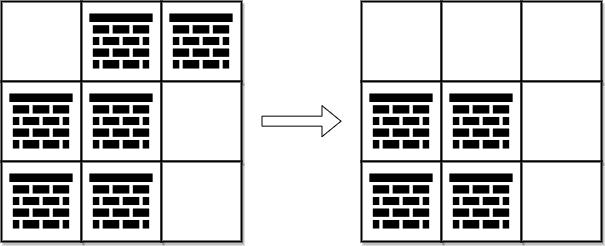## 战局详情

3944 / 6640Juruoer30:08:13

## 题目及解答

2287. 重排字符形成目标字符串

s 中取出字符并重新排列，返回可以形成 target最大 副本数。

• 1 <= s.length <= 100
• 1 <= target.length <= 10
• starget 由小写英文字母组成

2288. 价格减免

• 1 <= sentence.length <= 1e5
• sentence 由小写英文字母、数字、' ''$' 组成 • sentence 不含前导和尾随空格 • sentence 的所有单词都用单个空格分隔 • 所有价格都是 整数且不含前导零 • 所有价格 最多10 位数字 • 0 <= discount <= 100 示例一： 输入：sentence = “there are$1 $2 and 5$ candies in the shop”, discount = 50

2289. 使数组按非递减顺序排列

• 1 <= nums.length <= 1e5
• 1 <= nums[i] <= 1e9

• 步骤 1 ：[5,3,4,4,7,3,6,11,8,5,11] 变为 [5,4,4,7,6,11,11]
• 步骤 2 ：[5,4,4,7,6,11,11] 变为 [5,4,7,11,11]
• 步骤 3 ：[5,4,7,11,11] 变为 [5,7,11,11]
[5,7,11,11] 是一个非递减数组，因此，返回 3 。

nums[i] 若被删除，则一定是被 nums[i] 左边的某个值删除，可以证明， nums[i] 若被删除，则其被删除所需的步数可以等价于被其左边第一个大于 nums[i] 的值（设为 nums[j]）删除所需的步数。

[20,1,9,1,2,3] 为例。

• dp[i] = max(dp[j + 1], dp[j + 2], ... , dp[i - 1]) + 1

6081. 到达角落需要移除障碍物的最小数目

• 0 表示一个 单元格，
• 1 表示一个可以移除的 障碍物

• m == grid.length
• n == grid[i].length
• 1 <= m, n <= 1e5
• 2 <= m * n <= 1e5
• grid[i][j]01
• grid == grid[m - 1][n - 1] == 0Dijkstra 算法是很有名的算法了，不作过多解释，这里使用一个小根堆来维护待计算的点。

bfs 可以解决所有路径花销为 1 的图的最短理解问题，而 0-1bfs 则可以解决所有路径花销为 01 的图的最短路径问题。